October 10, 2022

## 一些概念

### 卷积

$$f(x)*g(x) = \displaystyle \int^{\infty}_{-\infty}f(\tau)g(x-\tau)d\tau$$

$$(f*g)(m,n)= \sum_i \sum_j f(i,j)g(m-i,n-j)$$

### 神经网络

#### M-P神经元模型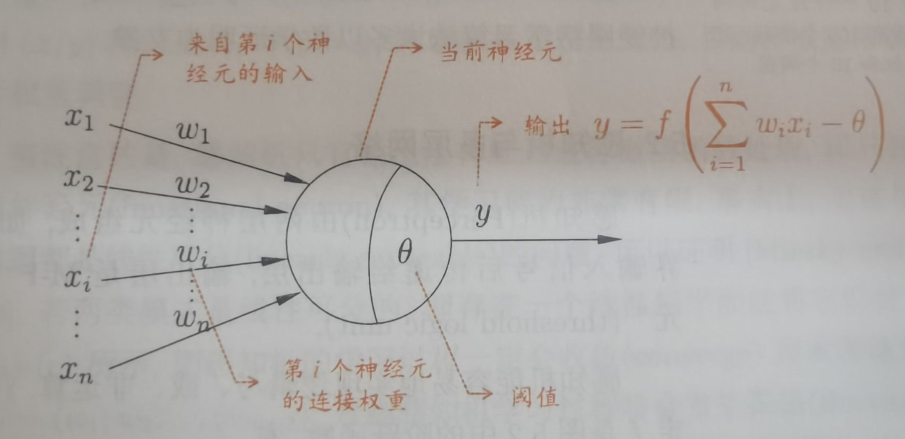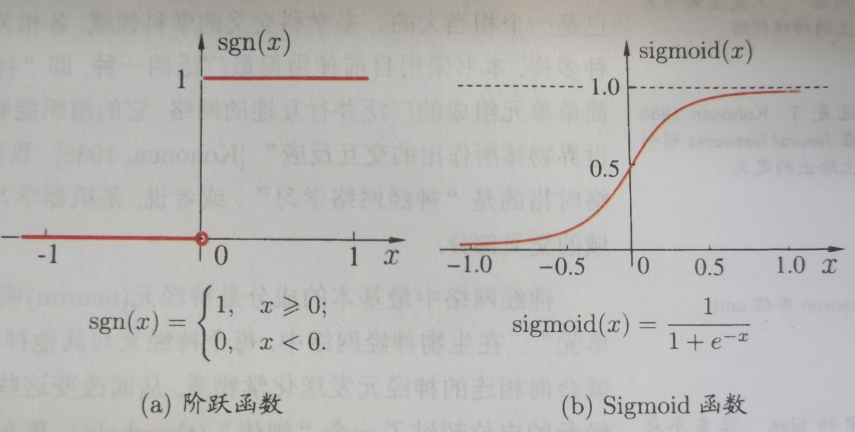### CNN(Convolutional Neural Network 卷积神经网络)

CNN的核心在于它的kernel，kernel是一个个小窗口，在图片上平移，通过卷积的方式来提取特征。这里的关键在于图片结构上的平移不变性：一个小窗口无论移动到图片的哪一个位置，其内部的结构都是一模一样的，因此CNN可以实现参数共享。

CNN中的卷积本质上就是利用一个共享参数的过滤器（kernel），通过计算中心像素点以及相邻像素点的加权和来构成feature map实现空间特征的提取，加权系数就是卷积核的权重系数。

### Graph embedding（图嵌入）

#### 图分析任务

• 节点分类
基于其他标记的节点和网络拓扑来确定节点的标签(也称为顶点)。
• 链接预测
预测缺失链路或未来可能出现的链路的任务。
• 聚类
发现相似节点的子集，并将它们分组在一起。
• 可视化
有助于深入了解网络结构。

#### 说明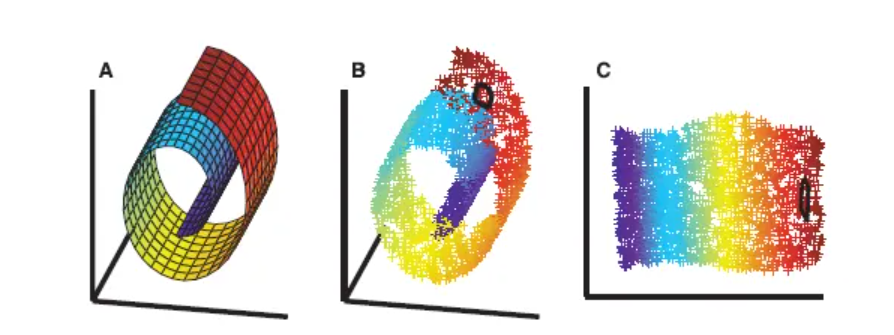#### 图嵌入的挑战

• 属性选择
节点的“良好”向量表示应保留图的结构和单个节点之间的连接。第一个挑战是选择嵌入应该保留的图形属性。考虑到图中所定义的距离度量和属性过多，这种选择可能很困难，性能可能取决于实际的应用场景。
• 可扩展性
大多数真实网络都很大，包含大量节点和边。嵌入方法应具有可扩展性，能够处理大型图。定义一个可扩展的模型具有挑战性，尤其是当该模型旨在保持网络的全局属性时。
• 嵌入的维数
实际嵌入时很难找到表示的最佳维数。例如，较高的维数可能会提高重建精度，但具有较高的时间和空间复杂性。较低的维度虽然时间、空间复杂度低，但无疑会损失很多图中原有的信息。

### 一些线代定义

#### 度矩阵(degree matrix)

$A=D^{-1}S$，其中$D$是度矩阵，$S$是邻接矩阵。

#### 特征矩阵

$\lambda E-A$为A的特征矩阵

#### 拉普拉斯矩阵

• $L=D-A$(度矩阵 $-$ 邻接矩阵)

### 归一化

#### 何时使用？

• 对输出结果范围有要求
• 数据较为稳定，不存在极端的最大最小值
• 数据存在异常值和较多噪音，用标准化，可以间接通过中心化避免异常值和极端值的影响。

### 几个学习概念

• 主动学习（active learning）
通过机器学习的方法获取到那些比较“难”分类的样本数据，让人工再次确认和审核，然后将人工标注得到的数据再次使用有监督学习模型或者半监督学习模型进行训练，逐步提升模型的效果，将人工经验融入机器学习的模型中。

• 监督学习（supervised learning ）
用一部分已知分类、有标记的样本来训练机器后，让它用学到的特征，对没有还分类、无标记的样本进行分类、贴标签。

• 半监督学习（semi-supervised learning）
有两个样本集，一个有标记，一个没有标记。综合利用有标记的样本和没有标记的样本，来生成合适的分类函数。

• 无监督学习（unsupervised learning ）
把相似度高的东西放在一起，对于新来的样本，计算相似度后，按照相似程度进行归类。

• 归纳学习（inductive learning）
训练集与测试集互斥

• 直推学习（transductive learning）
测试集（不带标签）包含在训练集中。有新样本时，需要重新计算。对于图而言，就是有新节点加入时，需要整张图重新计算。

## GCN(Graph Convolutional Network 图卷积网络)

### 来源

#### Spatial domain

• 每个顶点提取出来的neighbors不同，计算处理必须针对每个顶点
• 提取特征的效果可能没有卷积好

### 简单理解

#### 0x00 定义

• 每个节点$i$有一个特征值$x_i$，生成一个特征矩阵$X$（一个$N \times D$维的矩阵），其中$N$是节点个数，$D$是输入的特征数。
• 一个描述各个节点关系（图的结构）的矩阵，通常是邻接矩阵$A$

#### 0x01 举个例子

$$f(H^{(l)},A)= \sigma \big (AH^{(l)}W^{(l)}\big )$$
$W^{(l)}$是第$l$层神经网络层的系数矩阵，$\sigma(·)$是一个非线性激活函数（如$ReLU$）。虽然这个模型很简单，但它已经很强大。

• 只使用$A$的话，由于$A$的对角线上都是0，所以在和特征矩阵$H$相乘的时候，只会计算一个node的所有邻居的特征的加权和，该node自己的特征却被忽略了。因此，我们可以做一个小小的改动，给$A$加上一个单位矩阵$I$ ，这样就让对角线元素变成1了。
• $A$是没有经过归一化的矩阵，这样与特征矩阵相乘会改变特征原本的分布，产生一些不可预测的问题，比如邻居节点多的节点倾向于有更大的影响力。所以我们对$A$做一个标准化处理。首先让$A$的每一行加起来为1，我们可以乘以一个$D$的逆，$D$就是度矩阵。我们可以进一步把D的拆开与$A$相乘，得到一个对称且归一化的矩阵$D^{- \frac{1}{2}}AD^{- \frac{1}{2}}$

$H^{(l)}W^{(l)}$相当于给$l$层的所有节点的embedding做了一次线性变换。

#### 0x10 空手道俱乐部的embedding

GCN为什么这么强？

• 得到${ v_j }$的邻居节点的特征值${ h_{v_j} }$
• 更新节点特征$h_{v_j} \leftarrow hash \big (\sum_j h_{v_j}\big )$，理想情况下，$hash(·)$是一个injective hash function（简而言之，此哈希不能找到碰撞）

$$h_{v_i}^{(l+1)}= \sigma \bigg( \displaystyle \sum_{j} \frac{1}{c_{ij}} h^{(l)}_{v_j}W^{(l)} \bigg)$$

$j$是节点$v_i$的邻居节点的索引，$c_{ij}$是边$\big (v_i,v_j\big )$的归一化常量，它源于我们在GCN模型中使用的对称归一化邻接矩阵$D^{-\frac{1}{2}}AD^{-\frac{1}{2}}$。我们现在看到，这个传播规则可以被解释为可微分和参数化的（因为$W^{(l)}$是原始Weisfeiler-Lehman算法中使用的哈希函数的变体）。如果我们现在选择一个适当的非线性并初始化随机权重矩阵，使其正交，则这个规则会在实践中变得更稳定（也要归功于$c_{ij}$）。

### 缺陷

• GCN需要将整个图放到内存，比较耗内存，不能处理大图。
• 在训练时需要知道整个图的结构信息（包括待预测的节点）

## GraphSAGE

### 来源

GCN输入了整个图，训练节点收集邻居节点信息的时候，用到了测试和验证集的样本，我们把这个称为Transductive learning。

GraphSAGE的关键思想在于如何从节点的本地邻域（如邻居节点的度数或文本属性）聚合特征信息。

### 简单理解

GraphSAGE是一个Inductive Learning框架，具体实现中，训练时它仅仅保留训练样本到训练样本的边，然后包含Sample和Aggregate两大步骤

• Sample
对邻居的个数进行采样
• Aggregate
拿到邻居节点的embedding之后如何汇聚这些embedding以更新自己的embedding信息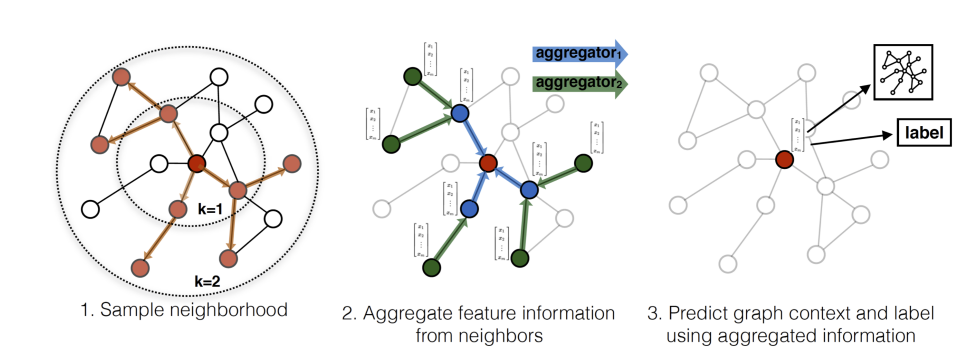1. 对邻居采样
2. 采样后的邻居embedding传到节点上来，并使用一个聚合函数聚合这些邻居信息以更新节点的embedding
3. 根据更新后的embedding预测节点的标签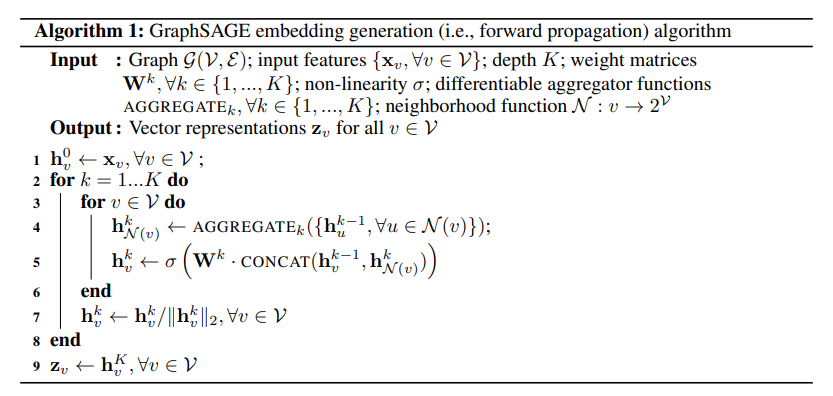#### 聚合器

##### Mean Aggregator

$h_v^k \leftarrow \sigma(W·MEAN({h_v^{k-1}} \cup {h_u^{k-1}, \forall u \in N(v)}))$

##### LSTM Aggregator

Applies LSTM to a random permutation of neighbors.

##### Pooling Aggregator

$AGGREGATE_k^{pool}=max({ \sigma (W_{pool}h_{u_i}^k +b) })$

### 总结

#### 优点

• 利用采样机制，解决了GCN必须要知道全部图的信息问题，克服了GCN训练时内存和显存的限制。即使对于未知的新节点，也能得到其表示
• 聚合器和权重矩阵的参数对于所有的节点是共享的
• 模型的参数的数量与图的节点个数无关，这使得GraphSAGE能够处理更大的图
• 既能处理有监督任务也能处理无监督任务

#### 缺点

• 没有考虑到不同邻居节点的重要性不同
• 聚合计算的时候邻居节点的重要性和当前节点也是不同的

## GAT

### 简单理解

Input features: $h={ \vec{h_1}, \vec{h_2} ,…,\vec{h_N} }$
Importance of $v_i$ to $v_i$: $e_{ij}=a(W \vec{h}_i,W \vec{h}_j)$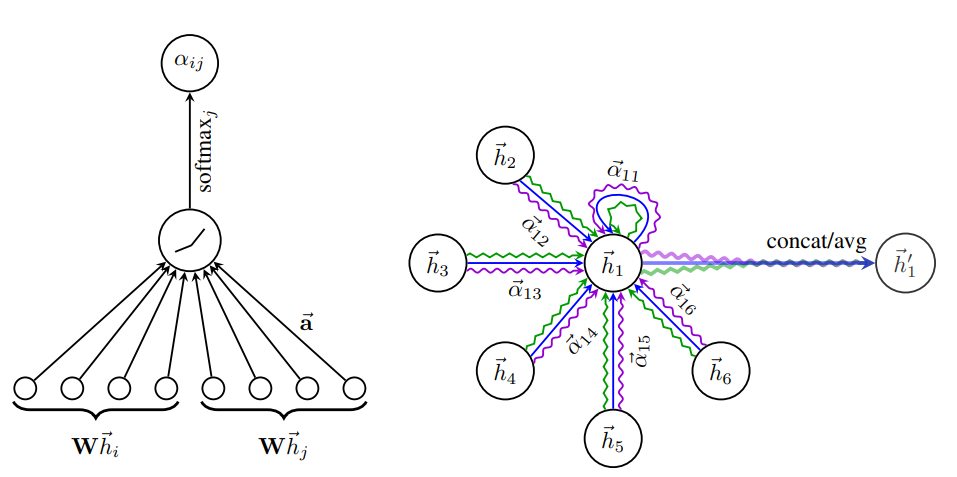$$\alpha_{ij}= \displaystyle \frac{exp \Big (LeakyReLU \Big ( \vec{a}^T[W \vec{h}_i] |W \vec{h}j \Big ) \Big )}{\sum{k \in N_i}exp \Big (LeakyReLU \Big ( \vec{a}^T[W \vec{h}_i] | W \vec{h}_k \Big ) \Big )}$$

$$\vec{h}i’= \sigma \Big ( \displaystyle \sum{j \in N_i}{\alpha_{ij}W \vec{h}_j} \Big )$$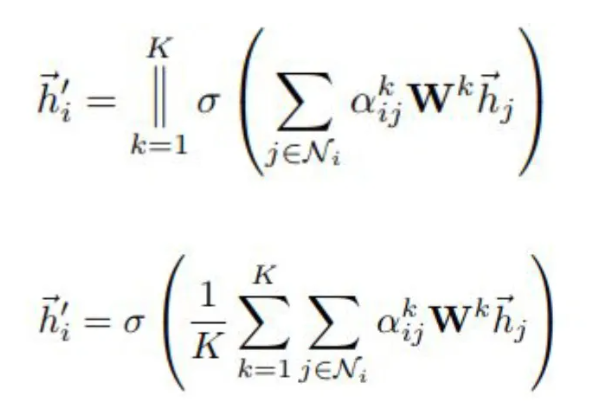### 优点

• 训练GCN无需了解整个图结构，只需知道每个节点的邻居节点即可
• 计算速度快，可以在不同的节点上进行并行计算
• 既可以用于Transductive Learning，又可以用于Inductive Learning，可以对未见过的图结构进行处理